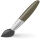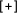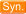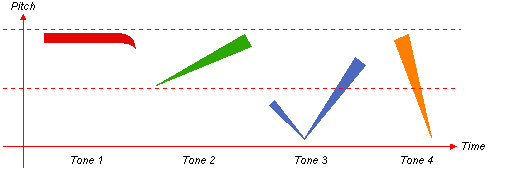# "Graph"  Chinese-English DictionaryDICTIONARY OPTIONS
 图形 tú xíng picture / figure / diagram / graph / depiction / graphical示意图 shì yì tú sketch / schematic diagram / graph图纸 tú zhǐ blueprint / drawing / design plans / graph paper均线 jūn xiàn graph of average values平面图 píng miàn tú a plan / a planar graph / a plane figure曲线图 qū xiàn tú line graph / line diagram线图 xiàn tú line drawing / diagram / line graph图论 tú lún graph theory (math.)展开图 zhǎn kāi tú expansion plan / development graph方格纸 fāng gé zhǐ squared paper / graph paper / grid paper (manuscript paper with squares for Chinese characters)柱型图 zhù xíng tú bar graph / bar diagram生成树 shēng chéng shù spanning tree (in graph theory)闭图象定理 bì tú xiàng dìng lǐ closed graph theorem (math)数字线划图 digital line graph柱形图表 bar graph / bar chart